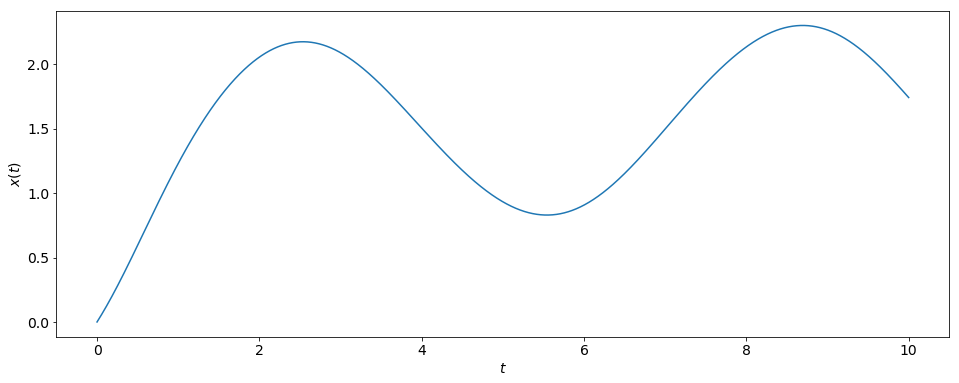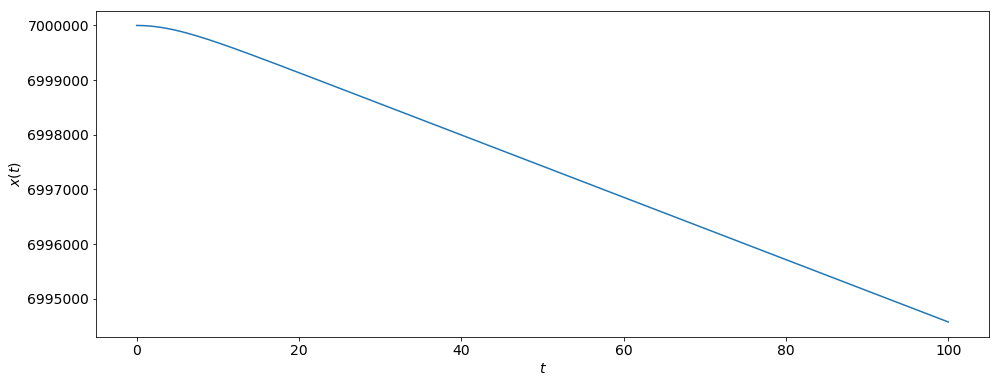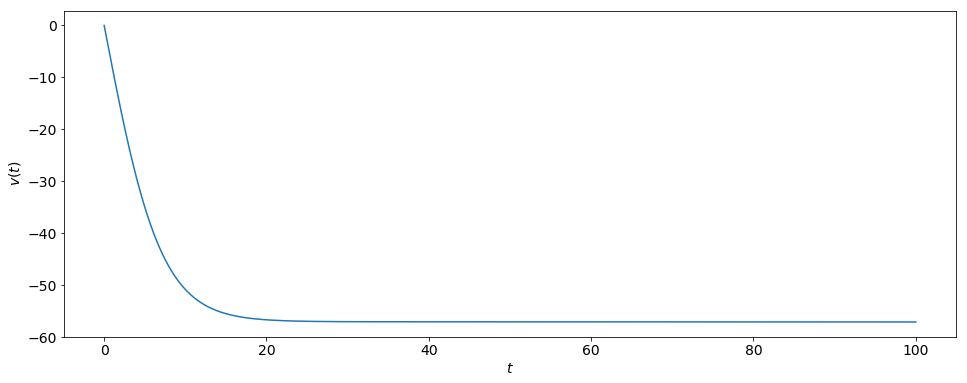# Euler's Method¶

### Modules - Ordinary Differential Equations¶

Last edited: March 11th 2018

Question:

How can we solve a first-order differential equation of the form $$\frac{d}{dt}x(t)=g(x(t),t),$$ with the initial condition $x(t_0)=x_0$, if we cannot solve it analytically?

Example 1:

We want to solve the ordinary differential equation (ODE) \begin{equation} \frac{d}{dt}x(t)=\cos(x(t))+\sin(t)\qquad\qquad\qquad\qquad \label{eq:1} \end{equation} with $x(0)=0$, i.e. we need to find the right function $x(t)$ that fulfills the ODE and the initial condition (IC).

Given the initial condition $x(0)=0$, we want to know $x(t)$ for $t>0$. We will now find an approximate numerical solution of the exact solution by computing the values of the function only at discrete values of $t$.

To do so, we define a discrete set of $t$-values, called grid points, by

$$t_n=t_0+n\cdot h~~~~~\mathrm{with}~~~~n=0,1,2,3,...,N.$$

The distance between two adjacent grid points is $h$. The largest value is $t_N=t_0+N*h$. Depending on the problem, $t_N$ might be given and $h$ is then determined by how many grid points $N$ we choose

$$h=\frac{t_N-t_0}{N}.$$

The key is now to approximate the derivative of $x(t)$ at a point $t_n$ by

\begin{equation} \frac{dx}{dt}_{t=t_n}\approx \frac{x(t_{n+1})-x(t_n)}{h},~~~~~h>0. \label{eq:2} \end{equation}

We know that this relation is exact in the limit $h\to 0$, since $x(t)$ is differentiable according to equation \eqref{eq:1}. For $h>0$, however, equation \eqref{eq:2} is only an approximation that takes into account the current value of $x(t)$ and the value at the next (forward) grid point. Hence, this method is called a forward difference approximation.

In equation \eqref{eq:2}, we approximate the slope of the tangent line at $t_n$ ("the derivative") by the slope of the chord that connects the point $(t_n,x(t_n))$ with the point $(t_{n+1},x(t_{n+1}))$. This is illustrated in the figure below: blue - graph; dotted - tangent line; green - chord.Substituting the approximation \eqref{eq:2} into \eqref{eq:1}, we obtain

\begin{equation*} \frac{x(t_{n+1})-x(t_n)}{h} \approx \cos(x(t_n))+\sin(t_n). \end{equation*}

Rearranging the equation, using the notation $x_n=x(t_n)$ and writing this as an equality (rather than an approximation) yields

$$x_{n+1} = x_n + h\left[ \cos(x_n)+\sin(t_n)\right].$$

This describes an iterative method to compute the values of the function successively at all grid points $t_n$ (with $t_n>0$), starting at $t_0=0$ and $x_0=0$ in our case. This is called Euler's method.

For example, the value of $x$ at the next grid point, $t_1=h$, after the starting point is

\begin{eqnarray*} x_{1} &=& x_0 + h\left[ \cos(x_0)+\sin(t_0)\right] \\ &=& 0 + h\left[ \cos(0) +\sin(0) \right] \\ &=& h. \end{eqnarray*}

Similarly, we find at $t_2=2h$

\begin{eqnarray*} x_{2} &=& x_1 + h\left[ \cos(x_1)+\sin(t_1)\right] \\ &=& h + h\left[ \cos(h) +\sin(h) \right] . \end{eqnarray*}

It is now a matter of what value to choose for $h$. To look at this, we will write some code which uses Euler's method to calculate $x(t)$.

In :
%matplotlib inline
import numpy as np
import matplotlib.pyplot as plt

# Set common figure parameters
newparams = {'figure.figsize': (16, 6), 'axes.grid': True,
'lines.linewidth': 1.5, 'lines.markersize': 10,
'font.size': 14}
plt.rcParams.update(newparams)

In :
N = 10000  # number of steps
h = 0.001  # step size

# initial values
t_0 = 0
x_0 = 0

t = np.zeros(N+1)
x = np.zeros(N+1)
t = t_0
x = x_0

t_old = t_0
x_old = x_0

for n in range(N):
x_new = x_old + h*(np.cos(x_old)+np.sin(t_old))  # Euler's method

t[n+1] = t_old+h
x[n+1] = x_new

t_old = t_old+h
x_old = x_new

print(r'x_N = %f' % x_old)

# Plot x(t)
plt.figure()
plt.plot(t, x)
plt.ylabel(r'$x(t)$')
plt.xlabel(r'$t$')
plt.grid()
plt.show()

x_N = 1.742849In the code above, we have chosen $h=0.001$ and $N=10000$, and so $t_N=10$. In the plot of $x(t)$, the discrete points have been connected by straight lines.

What happens to $x_N$ when we decrease $h$ by a factor of $10$? (Remember to increase $N$ simultaneously by a factor of $10$ so as to obtain the same value for $t_N$.)

#### Accuracy:¶

We see that the value of $x_N$ depends on the step size $h$. In theory, a higher accuracy of the numerical solution in comparison to the exact solution can be achieved by decreasing $h$ since our approximation of the derivative $\frac{d}{dt}x(t)$ becomes more accurate.

However, we cannot decrease $h$ indefinitely since, eventually, we are hitting the limits set by the machine precision. Also, lowering $h$ requires more steps and, hence, more computational time.

For Euler's method, it turns out that the global error (error at a given $t$) is proportional to the step size $h$ while the local error (error per step) is proportional to $h^2$. This is called a first-order method.

We can now summarize Euler's method.

Given the ODE $$\frac{d}{dt}x(t)=g(x(t),t)~~~\mathrm{with}~~~x(t_0)=x_0,$$ we can approximate the solution numerically in the following way:

1. Choose a step size $h$.
2. Define grid points: $t_n=t_0+n*h~~\mathrm{with}~~n=0,1,2,3,...,N$.
3. Compute iteratively the values of the function at these grid points: $x_{n+1}=x_n+h*g(x_n,t_n)$. Start with $n=0$.

#### Instability:¶

Apart from its fairly poor accuracy, the main problem with Euler's method is that it can be unstable, i.e. the numerical solution can start to deviate from the exact solution in dramatic ways. Usually, this happens when the numerical solution grows large in magnitude while the exact solution remains small.

A popular example to demonstrate this feature is the ODE $$\frac{dx}{dt}=-x~~~\mathrm{with}~~~x(0)=1.$$ The exact solution is simply $x(t)=e^{-t}$. It fulfills the ODE and the initial condition.

On the other hand, our Euler method reads $$x_{n+1}=x_n+h\cdot(-x_n)=(1-h)x_n.$$

Clearly, if $h>1$, $x(t_n)$ will oscillate between negative and positive numbers and grow without bounds in magnitude as $t_n$ increases. We know that this is incorrect since we know the exact solution in this case.

On the other hand, when $0<h<1$, the numerical solution approaches zero as $t_n$ increases, reflecting the behavior of the exact solution.

Therefore, we need to make sure that the step size of the Euler method is sufficiently small so as to avoid such instabilities.

### Second Order ODEs¶

We will now demonstrate how Euler's method can be applied to second-order ODEs.

In physics, we often need to solve Newton's law which relates the change in momentum of an object to the forces acting upon it. Assuming constant mass, it usually has the form $$m\frac{d^2}{dt^2}x(t)=F(v(t),x(t),t),$$ where we restrict our analysis to one dimension. (The following ideas can be extended to two and three dimensions in a straightforward manner.)

Dividing by the mass, we find $$\frac{d^2}{dt^2}x(t)=G(v(t),x(t),t),$$ with $G(v,x,t)\equiv F(v,x,t)/m$. We can re-write this second-order ODE as two coupled, first-order ODEs. By definition, we have $v(t)=\frac{d}{dt}x(t)$. Hence, we obtain \begin{eqnarray*} \frac{dx}{dt} &=& v, \\ \frac{dv}{dt} &=& G(v,x,t). \end{eqnarray*} Now, we only need to specify the initial conditions $x_0=x(t_0)$ and $v_0=v(t_0)$ to have a well-defined problem.

Using the same discretization of time as previously, we can apply the ideas of Euler's method also to this first-order system. It yields \begin{eqnarray*} x_{n+1} &=& x_n+h\cdot v_n, \\ v_{n+1} &=& v_n+h\cdot G(v_n,x_n,t_n), \end{eqnarray*}
where (at $n=0$) $x_0$ and $v_0$ are the initial conditions at $t=t_0$.

Example 2:

Let us consider a particle of mass $m$ that is in free fall towards the center of a planet of mass $M$. Let us assume that the atmosphere exerts a force $$F_\mathrm{drag}=Dv^2$$ onto the particle which is proportional to the square of the velocity. Here, $D$ is the drag coefficient. Note that the $x$-axis is pointing away from the planet. Hence, we only consider $v\leq 0$.

The particle motion is described by the following governing equation ($G$: gravitational constant) $$m\frac{d^2 x}{dt^2}=Dv^2-\frac{GmM}{x^2}.$$

Dividing each side by $m$ gives $$\frac{d^2 x}{dt^2}=\frac{D}{m}v^2-\frac{GM}{x^2}.$$ Following our recipe above, we re-cast this as two first-order ODEs \begin{eqnarray*} \frac{dx}{dt} &=& v, \\ \frac{dv}{dt} &=& \frac{D}{m}v^2-\frac{GM}{x^2}. \end{eqnarray*} We choose $D=0.0025\,\mathrm{kg}\,\mathrm{m}^{-1}$, $m=1\,\mathrm{kg}$ and $M=M_\mathrm{Earth}$, i.e. the mass of the Earth.

Accordingly, our algorithm now reads \begin{eqnarray*} x_{n+1} &=& x_n+h*v_n, \\ v_{n+1} &=& v_n+h*\left[ \frac{D}{m}v_n^2-\frac{GM}{x_n^2} \right] . \end{eqnarray*}
Let us specify the following initial conditions and step size: $$t_0=0,~~x(t_0)=x_0=7000.0\,\mathrm{km},~~v(t_0)=v_0 =0\,\mathrm{m/s},~~h=0.001\,\mathrm{s}.$$ We could now iterate the above equations until the particle hits the ground, i.e. until $x=R_\mathrm{Earth}$, where $R_\mathrm{Earth}$ is the radius of Earth. This occurs in finite time both in reality and in our code.

Moreover, the particle would also reach $x=0$ in finite time, given the above equations, while the speed grows to infinity. However, the code would crash well before $x$ approaches zero due to the speed reaching very large values.

Note: The governing equation actually changes when $|x|<R$.

Therefore, we need to be careful with our numerical solution procedure. This goes to show that it is often very useful to understand the physical problem under consideration when solving its governing equations numerically.

Let us integrate until $t_N=100\,\mathrm{s}$, equivalent to $N=10^5$ time steps. As it turns out, the particle does not reach the ground by $t=t_N$.

In :
G = 6.67300e-11 # gravitational constant
M = 5.97219e24  # mass of Earth
D = 0.0025      # drag coefficient
m = 1.0         # mass of particle

N = 100000      # number of steps
h = 0.001       # step size

# initial values
t_0 = 0.0
x_0 = 7.0e6
v_0 = 0.0

t = np.zeros(N+1)
x = np.zeros(N+1)
v = np.zeros(N+1)
t = t_0
x = x_0
v = v_0

for n in range(N):
# Euler's method
x_new = x[n] + h*v[n]
v_new = v[n] + h*((D/m)*v[n]**2-G*m*M/x[n]**2)

t[n+1] = t[n] + h
x[n+1] = x_new
v[n+1] = v_new

plt.figure()
plt.plot(t,x)  # plotting the position vs. time: x(t)
plt.xlabel(r'$t$')
plt.ylabel(r'$x(t)$')
plt.grid()

plt.figure()
plt.plot(t,v)  # plotting the velocity vs. time: v(t)
plt.xlabel(r'$t$')
plt.ylabel(r'$v(t)$')
plt.grid();What do you observe? What happens if you choose $x_0=10000.0\,\mathrm{km}$ as your initial height?

### Summary:¶

We have learned how to use Euler’s method to solve first-order and second-order ODEs numerically. It is the simplest method in this context and very easy to implement. However:

1. There are more precise methods for solving ODEs.
2. There are more stable methods for solving ODEs.

Notwithstanding these issues, Euler’s method is often useful: it is easy to use (the coding is less error prone); it can provide a helpful first impression of the solution; modern-day computer power makes computational expense less of an issue. Simply put, sometimes it is sufficient.